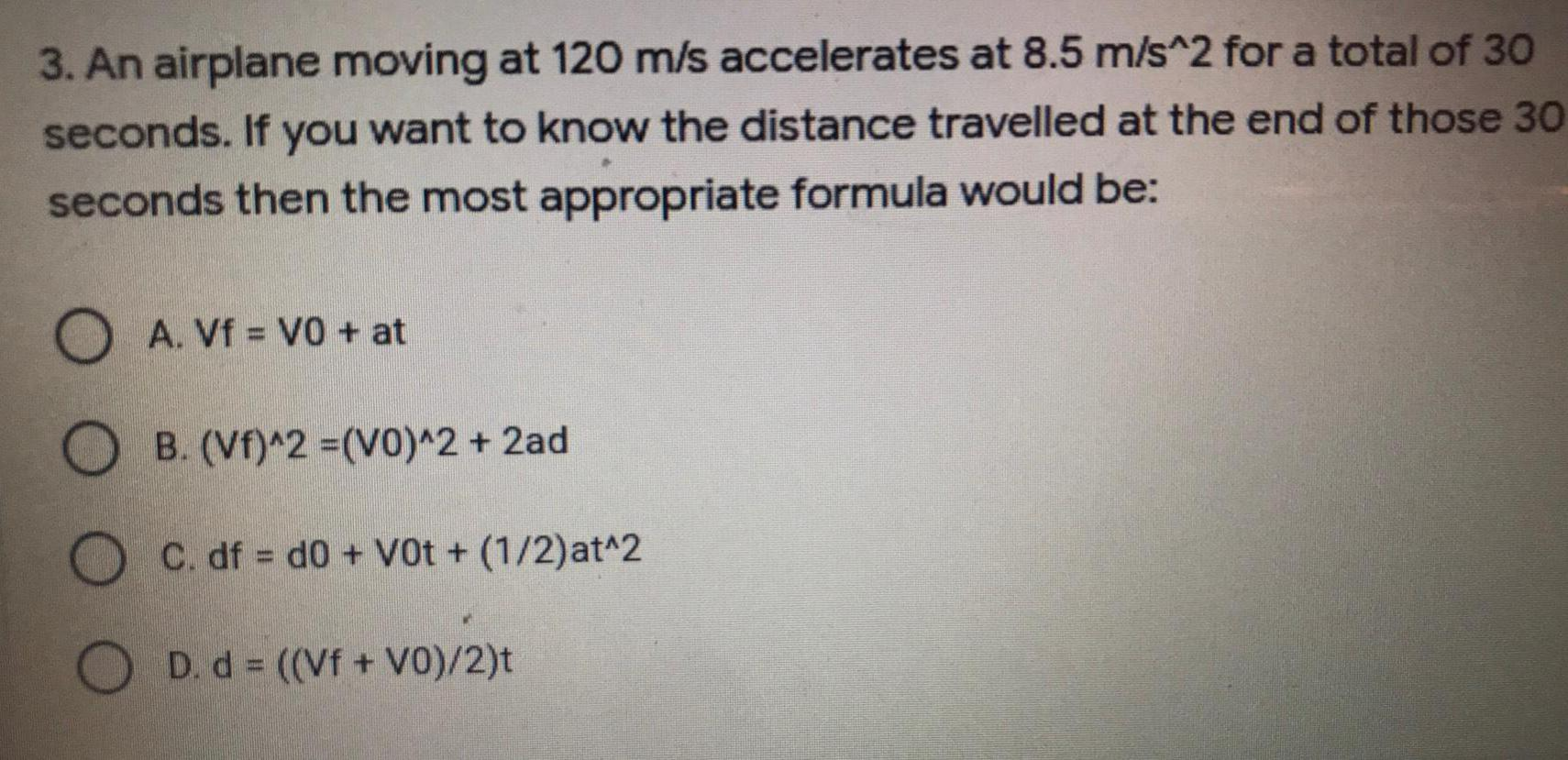Question:

# An airplane moving at 120 m/s accelerates at 8.5 m/s^2 for aAn airplane moving at 120 m/s accelerates at 8.5 m/s^2 for a total of 30 seconds. If you want to know the distance travelled at the end of those 30 seconds then the most appropriate formula would be: A. Vf = V0 + at B. (Vf)^2 =(VO)^2 + 2ad C. df = d0 +V0t + (1/2) at^2 D. d = ((Vf + V0)/2)t